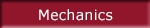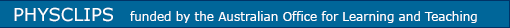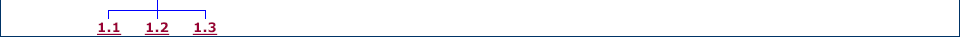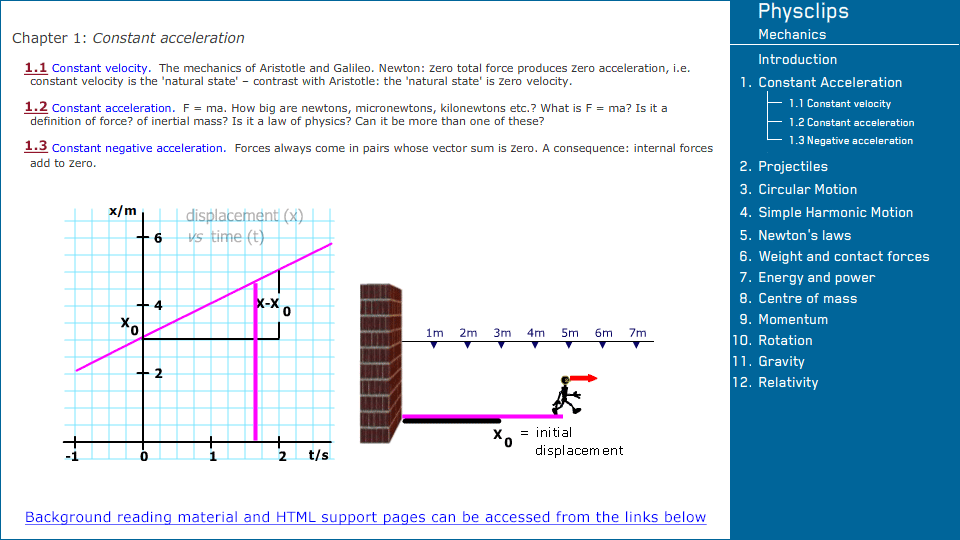Links to related material and teachers' resources A simple introduction to calculus: Differentials and integrals A simple, physics-based guide to quantities that vary: differentiation and integration, numerical and analytical, the log function, polynomials, trigonometric functions, the chain rule, techniques for integration, partial derivatives, introduction to logs Graphs, errors, dimensions and units Drawing graphs, handling units and errors, significant figures, the method of dimensions, dealing with non-SI units Vectors Vectors, vector components, unit vectors, two and three dimensions, vector addition and subtraction, relative motion and moving coordinates, the scalar product of vectors (the dot product), the vector product (the cross product) Kinematics Motion in one dimension under constant velocity or constant acceleration: deriving the equations from the definitions of velocity and acceleration. Analysing Motion Using frame grabbing software and spreadsheet software to determine velocity and accleration of an object. Using a spreadsheet Using a spreadsheet for repetitive data handling. Car Physics Acceleration examples: braking with constant force and decelerating with air resistance. Some other problems in car physics Download all animations in this chapter (or individually below each thumbnail) Constant Acceleration